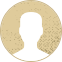Top Special Offer! Check discount

Get 13% off your first order - useTopStart13discount code now!

# Mean Deviation and Standard Deviation

214 views 3 pages ~ 577 words
Get a Custom Essay Writer Just For You!

Experts in this subject field are ready to write an original essay following your instructions to the dot!

In order to calculate the mean deviation (mean absolute deviation) and standard deviation, we first calculate the mean of the data set. The mean or average is given by the formula;

Mean=   . For Set A, Mean= 15/6 =2.5 while mean for set B = 15/6= 2.5. The two sets have equal means. The following tables summarize the calculations for range, mean deviation, and standard deviation for the two data sets.

A

x

x-ẋ

x-ẋ

[x-ẋ]2

4

1.5

1.5

2.25

6

3.5

3.5

12.25

2

-0.5

0.5

0.25

1

-1.5

1.5

2.25

1

-1.5

1.5

2.25

1

-1.5

1.5

2.25

Total

10

21.251

a) Range

The range shows the difference between the lowest and the highest values in the data set. In this case, the highest score is 6 and the lowest score is 1. Range= 6-1 = 5

b) Mean Deviation

Mean deviation gives the average of the absolute differences between actual observations from the mean. Mean deviation (mean absolute deviation) is given by the formula;

c) Standard Deviation

Standard deviation reflects a measure of dispersion of sample values from the sample mean. For ungrouped data, it is given by the formula;

δ =   = (21.251/5)1/2 = 2.0616

B

x

x-ẋ

x-ẋ

[x-ẋ]2

3

0.5

0.5

0.25

3

0.5

0.5

0.25

2

-0.5

0.5

0.25

1

-1.5

1.5

2.25

4

1.5

1.5

2.25

2

-0.5

0.5

0.25

Total

5

5.5

a) Range

The highest value is 4 while the lowest value is 1. The range for the data is 4-1 = 3.

b) Mean Deviation

Using the formula stated in part A above, the mean deviation is given by MAD= (5/6) = 0.8333

c) Standard Deviation

Replacing the computed values in the formula δ =  ,  we obtain, δ= (5.5/5)1/2 = 1.0488

Analysis

Comparing the variability of attitudes in the two classes clearly show that class A has higher variability than class B. The range for class A is 5 while class B has a range of 3. The mean deviation for the two classes is 1.6667 and 0.8333. Moreover, the standard deviations are 2.0616 and 1.0488. From the above data, it is evident that class A has varying attitudes towards segregation compared to class B. Large values of mean deviation, range, and standard deviation indicates the presence of higher variability in a set of data.

Question Two

The mean for a grouped data is given by Mean=  = 184/19= 9.6842

The table summarizes calculations of range, standard deviations, and mean deviations involving grouped data.

x

f

fx

x-ẋ

x-ẋ

f x-ẋ

[x-ẋ]2

f[x-ẋ]2

12

4

48

5.316

5.316

21.264

28.2599

113.0396

11

3

33

1.316

1.316

3.948

1.7319

5.1957

10

2

20

0.316

0.316

0.632

0.0999

0.1998

9

5

45

-0.684

0.684

3.42

0.4679

2.3395

8

3

24

-0.684

0.684

5.052

2.8359

8.5077

7

2

14

-2.684

2.684

5.368

7.2039

14.4078

Total

19

184

39.684

143.6901

From the calculations in the table above, the following can be derived;

a) Range

The maximum value is 12 while the lowest value is 7. The range for the data is (12-7)= 5.

b) Mean Deviation

The mean absolute deviation for a grouped data is given by the formula

MAD= Replacing the computed values in the formula, we obtain;

c) Standard Deviation

The standard deviation for a grouped data is given by the formula, δ =

δ =   = 2.8254

Question Three

In order to calculate the mean of the grouped data, we first compute the midpoints of the classes. The following formula is then used to calculate the mean; Mean=  . Where n is the cumulative frequency or the sum of corresponding frequencies.

Mean= 1843.5/23 = 80.1522

The table below shows a summary of the calculations used for calculations of the mean, mean deviation, and standard deviation for a grouped data.

Class

f

x

fx

x-ẋ

x-ẋ

f x-ẋ

[x-ẋ]2

f[x-ẋ]2

50-59

2

54.5

109

-25.6522

25.6522

51.3044

658.0354

1316.0708

60-69

3

64.5

193.5

-15.6522

15.6522

46.9566

244.9914

734.9742

70-79

4

74.5

298

-5.6522

5.6522

22.6088

31.9474

127.7896

80-89

8

84.5

676

4.3478

4.3478

34.7824

18.9034

151.2272

90-99

6

94.5

567

14.3478

14.3478

86.0868

205.8594

1235.1564

Total

23

1843.5

241.739

3565.2182

a) Range

Using the lower class limit of the lowest class and the highest-class limit of the highest class, we can obtain the range of the data. The range range is given by (99.5-49.5)= 50.

b) Mean Deviation

MAD for a grouped data is given by the formula,

MAD= . Replacing values from the table in the formula, we obtain;

c) Standard Deviation

Replacing computed values in the formula δ = , we obtain δ =

δ = 12.7301

September 18, 2023
Category:
Subcategory:
Subject area:
Number of pages

3

Number of words

577

62Rate:

4.7

Expertise Mean
Verified writer

Clive2020 is an excellent writer who is an expert in Nursing and Healthcare. He has helped me earn the best grades with a theorists paper and the shadowing journal. Great job that always stands out!

Hire Writer

This sample could have been used by your fellow student... Get your own unique essay on any topic and submit it by the deadline.

Eliminate the stress of Research and Writing!

Hire one of our experts to create a completely original paper even in 3 hours!